# 9.2.3 Loci in Two Dimensions, PT3 Focus Practice

Question 7:
Diagram below in the answer space shows a circle with centre O drawn on a grid of equal squares with sides of 1 unit. POQ is a diameter of the circle.
W, X and Y are three moving points inside the circle.
(a) W is the point which moves such that it is constantly 4 units from the point O. Describe fully the locus of W.
(b) On the diagram, draw,
(i) the locus of the point X which moves such that its distance is constantly 3 units from the line PQ,
(ii) the locus of the point Y which moves such that it is equidistant from the point P and the point Q.
(c) Hence, mark with the symbol $\otimes$ the intersection of the locus of X and the locus of Y.

(b)(i),(ii) and (c)Solution:
(a) The locus of W is a circle with the centre O and a radius of 4 units.

(b)(i),(ii) and (c)Question 8:
The diagram in the answer space shows two squares ABCD and CDEF each of sides 4 cm. K is a point on the line CD. W, X and Y are three moving points in the diagram.
(a) Point W moves such that it is always equidistant from the straight lines AB and EF. By using the letters in diagram, state the locus of W.
(b) On the diagram, draw
(i) the locus X such that it is always 2 cm from the straight line ACE,
(ii) the locus of Y such that KY = KC.
(c) Hence, mark with the symbol $\otimes$ the intersection of the locus of X and the locus of Y.

(b)(i), (ii) and (c)Solution:
(a) The locus of W is the line CD.

(b)(i),(ii) and (c)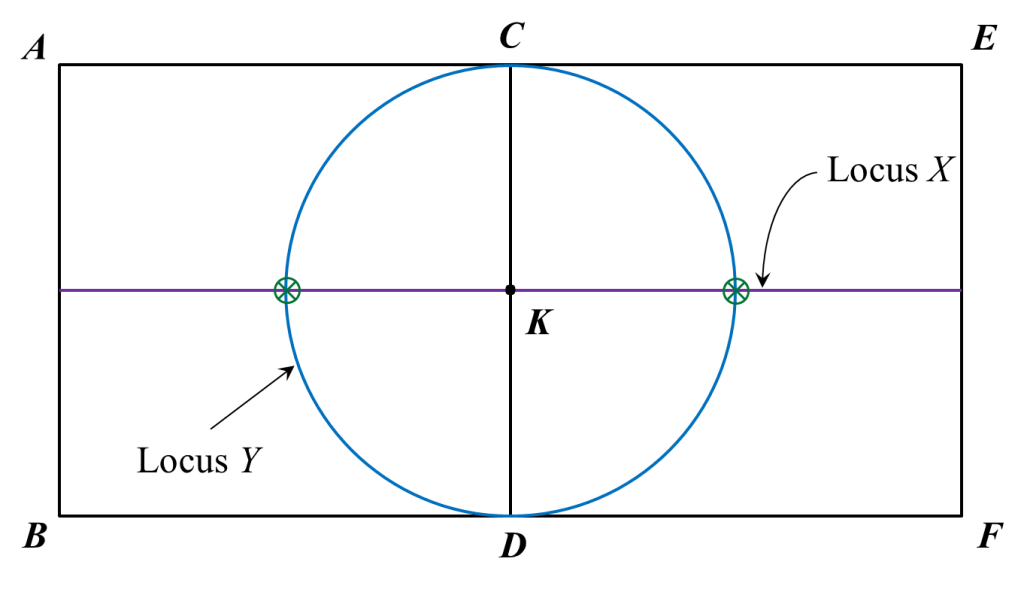Question 9:
Diagram below shows a Cartesian plane.
Draw the locus of X, Y and Z.
(a) X is a point which moves such that its distance is constantly 4 units from the origin.
(b) Y is a point which moves such that it is always equidistant from point K and point L.
(c) Z is a point which moves such that it is always equidistant from lines KM and y-axis.Solution: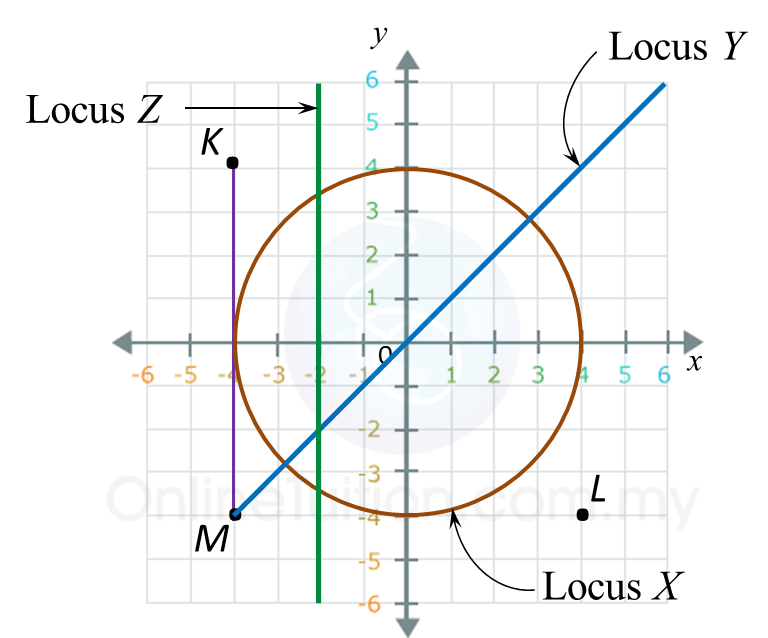# 9.2.2 Loci in Two Dimensions, PT3 Focus Practice

9.2.2 Loci in Two Dimensions, PT3 Focus Practice

Question 4:
Diagram below in the answer space shows a quadrilateral ABCD drawn on a grid of equal squares with sides of 1 unit.
X, Y and Z are three moving points inside the quadrilateral ABCD.
(a) X is the point which moves such that it is always equidistant from point B and point D.
By using the letters in diagram, state the locus of X.
(b) On the diagram, draw,
(i) the locus of the point Y such that it is always 6 units from point A,
(ii) the locus of the point Z which moves such that its distance is constantly 3 units from the
line AB.
(c) Hence, mark with the symbol ⊗ the intersection of the locus of Y and the locus of Z.

:
(b)(i),(ii) and (c)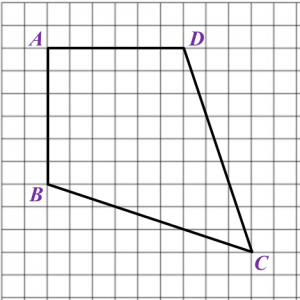Solution
:
(a) The locus of X is the line AC.

(b)(i),(ii) and (c)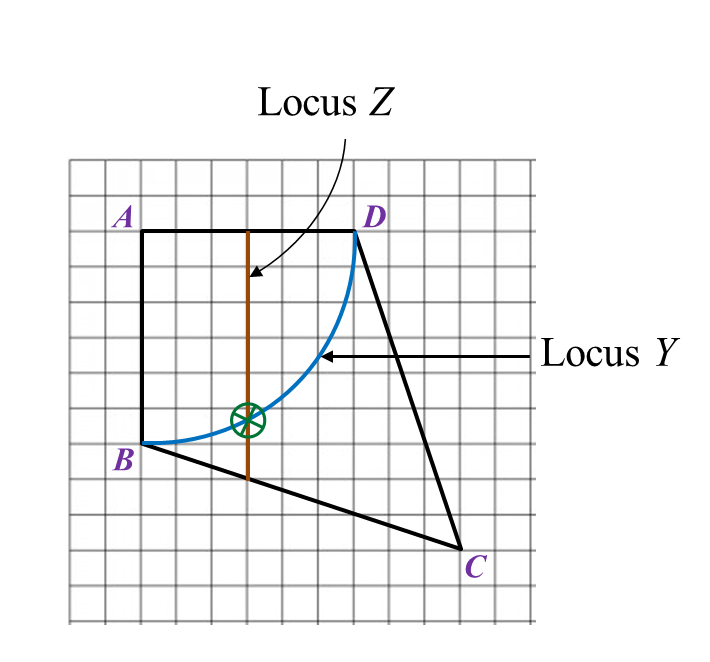Question 5:
Diagram in the answer space below shows a square ABCD. E, F, G and H are the midpoints of straight lines AD, AB, BC and CD respectively. W, X and Y are moving points in the square.
On the diagram,
(a) draw the locus of the point W which moves such that it is always equidistant from point AD and BC.
(b)   draw the locus of the point X which moves such that XM = MG.
(c) draw the locus of point Y which moves such that its distance is constantly 6 cm from point C.
(d)   Hence, mark with the symbol ⊗  the intersection of the locus of W and the locus of Y.

:
(a), (b), (c) and (d)Solution
: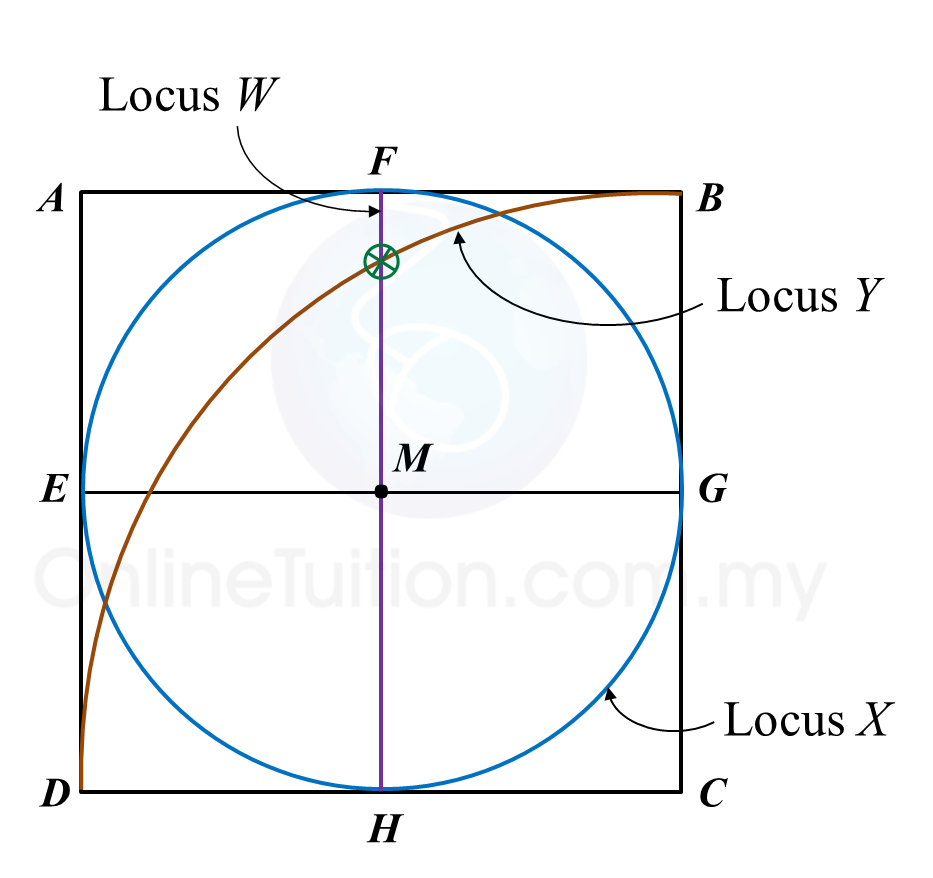Question 6:
Diagram in the answer space below shows a polygon ABCDEF drawn on a grid of squares with sides of 1 unit. X, Y and Z are the points that move in the polygon.
(a) X is a point which moves such that it is equidistant from point B and point F.
By using the letters in diagram, state the locus of X.
(b) On the diagram, draw
(i) the locus of the point Y which moves such that it is always parallel and equidistant from the straight lines BA and CD.
(ii) the locus of point Z which moves such that its distance is constantly 6 units from the point A.
(c) Hence, mark with the symbol $\otimes$ the intersection of the locus of Y and the locus of Z.

(b)(i), (ii) and (c)Solution:
(a) The locus of X is AD.

(b)(i),(ii) and (c)# 9.2.1 Loci in Two Dimensions, PT3 Focus Practice

9.2.1 Loci in Two Dimensions, PT3 Focus Practice

Question 1:
Diagram below in the answer space shows a square PQRS with sides of 6 units drawn on a grid of equal squares with sides of 1 unit. W, X and Y are three moving points inside the square.
(a) W is the point which moves such that it is always equidistant from point P and point R.
By using the letters in diagram, state the locus of W.
(b) On the diagram, draw,
(i) the locus of the point X which moves such that it is always equidistant from the straight lines PQ and PS,
(ii) the locus of the point Y which moves such that its distance is constantly 2 units from point K.
(c) Hence, mark with the symbol ⊗ the intersection of the locus of X and the locus of Y.

:
(b)(i),(ii)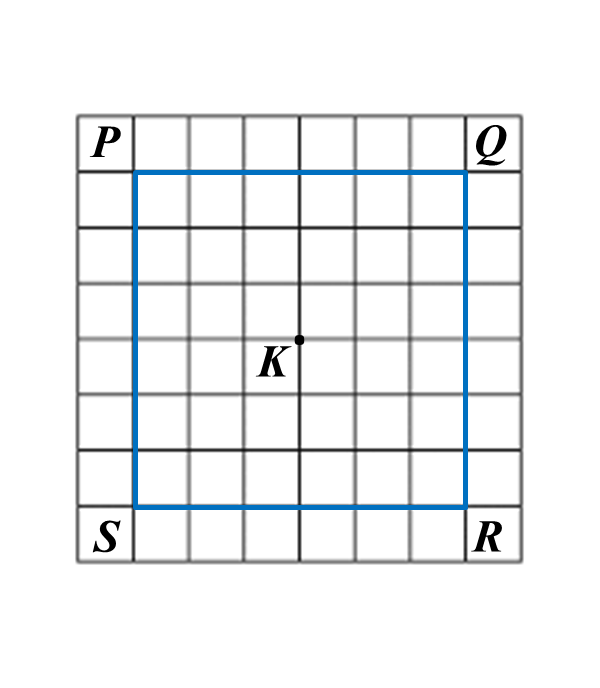Solution:
(a) QS

(b)(i),(ii)
(c)Question 2:
Diagram in the answer space below, shows a regular pentagon PQRST. W, X and Y are moving points which move in the pentagon.
On the diagram,
(a) draw the locus of the point W which moves such that it is always equidistant from point R and S.
(b) draw the locus of the point X which moves such that XR = RS.
(c) draw the locus of point Y which moves such that its distance is constantly 3 cm from the line SR.
(d) hence, mark with the symbol ⊗ the intersection of the locus of W and the locus of X.

:
(a), (b), (c) and (d)Solution:
(a), (b), (c) and (d)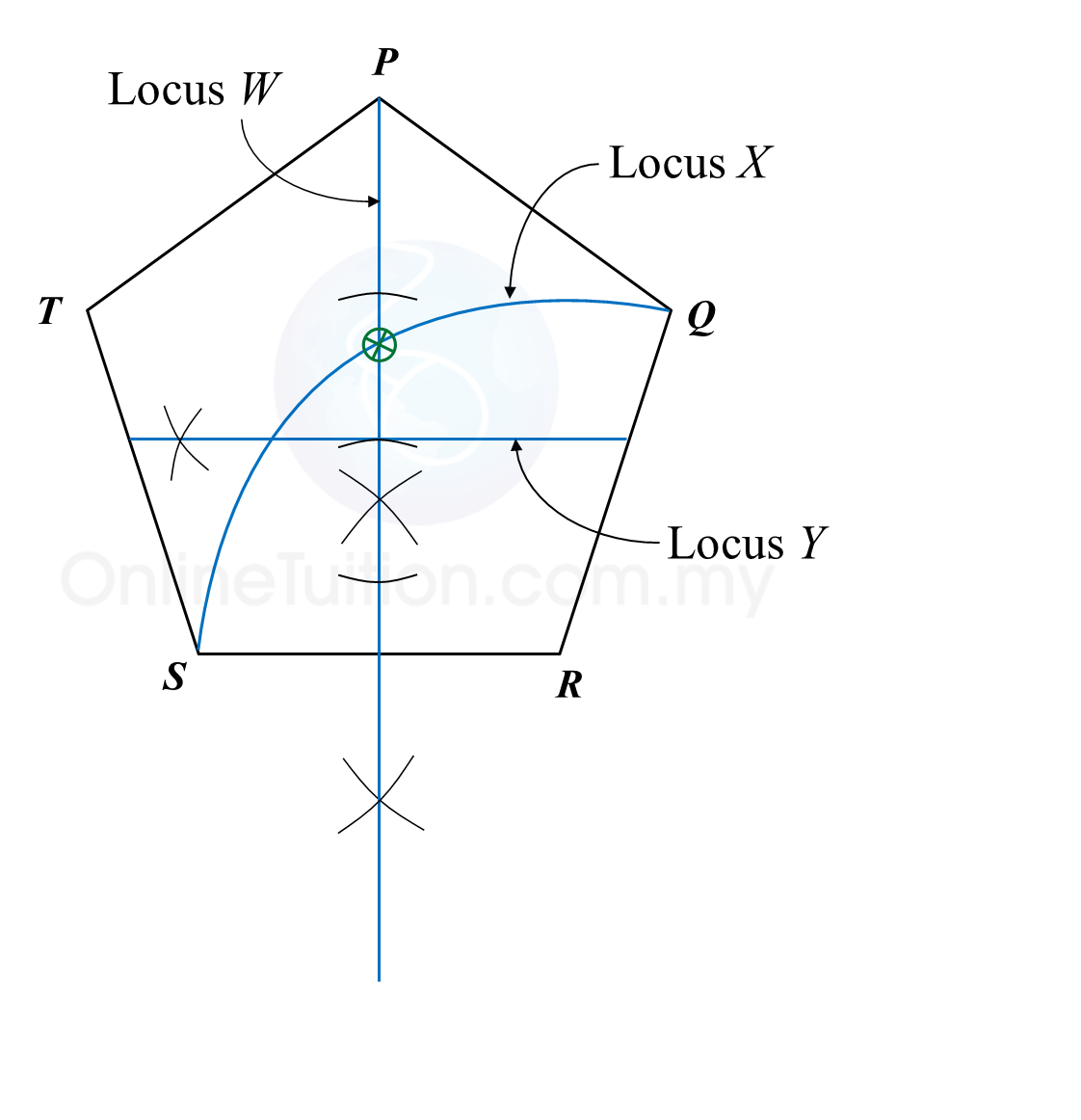Question 3:
Diagram in the answer space below shows a polygon. X and Y are two moving points in the polygon.
(a) On the diagram, draw
(i) the locus of the point X such that XQ = XR.
(ii) the locus of the point Y such that YQ = QR.
(b) Hence, mark with the symbol $\otimes$ the intersection of the locus of X and the locus of Y.